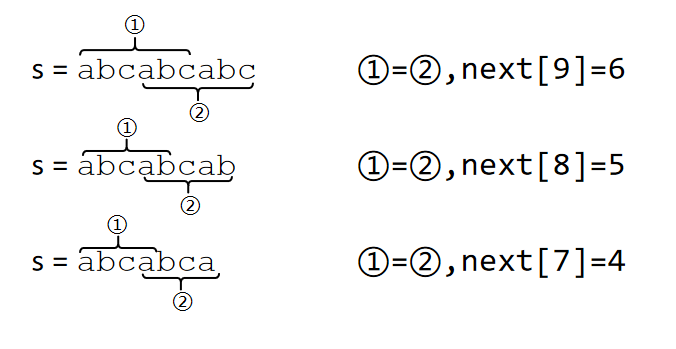# LOJ#10045. 「一本通 2.2 练习 1」Radio Transmission

UPD：更新了一种推导方法，但原推导方法没有删除。

1. 字符串下标从 $1$ 开始
2. $l$ 代表题目给定字符串 $s$ 的长度；
3. $\operatorname{next}$ 代表 KMP 算法的失配函数，$\operatorname{next}(i)$ 表示 $s_{1\dots i}$ 的最长公共前缀后缀的长度；
4. 对于两个字符串 $s_1,s_2$$s_1+s_2$ 代表将两个字符串拼接起来，如 $\mathtt{abc}+\mathtt{defg}=\mathtt{abcdefg}$

$s=\mathtt{abcabcabc}$，这时 $s_{1\dots 6}=s_{4\dots 9}$$\operatorname{next}(9)=6$#include<iostream>
#include<cstdio>
#include<algorithm>
#include<cstring>
using namespace std;
const int N=1e6;
char s[N+10];
int nxt[N+10],n;
void init()
{
memset(nxt,0,sizeof(nxt));
int n=strlen(s);
for(int i=1;i<n;i++)
{
int j=nxt[i-1];
while(j && s[i]!=s[j]) j=nxt[j-1];
if(s[i]==s[j]) j++;
nxt[i]=j;
}
}
int main()
{
scanf("%d%s",&n,s);
init();
printf("%d\n",n-nxt[n-1]);
return 0;
}

posted @ 2020-07-16 21:24  zzt1208  阅读(40)  评论(4编辑  收藏